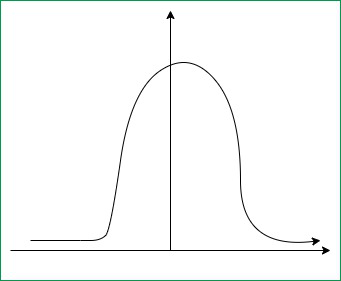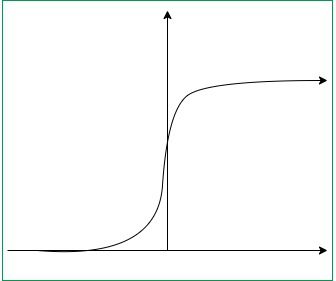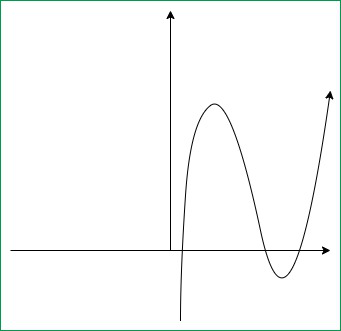# Major Kernel Functions in Support Vector Machine (SVM)

• Difficulty Level : Medium
• Last Updated : 16 Jul, 2020

Kernel Function is a method used to take data as input and transform into the required form of processing data. “Kernel” is used due to set of mathematical functions used in Support Vector Machine provides the window to manipulate the data. So, Kernel Function generally transforms the training set of data so that a non-linear decision surface is able to transformed to a linear equation in a higher number of dimension spaces. Basically, It returns the inner product between two points in a standard feature dimension.

Standard Kernel Function Equation :Attention reader! Don’t stop learning now. Get hold of all the important Machine Learning Concepts with the Machine Learning Foundation Course at a student-friendly price and become industry ready.

Major Kernel Functions :-

For Implementing Kernel Functions, first of all we have to install “scikit-learn” library using command prompt terminal:

    pip install scikit-learn

• Gaussian Kernel: It is used to perform transformation, when there is no prior knowledge about data.• Gaussian Kernel Radial Basis Function (RBF) : Same as above kernel function, adding radial basis method to improve the transformation.Code:
 from sklearn.svm import SVCclassifier = SVC(kernel ='rbf', random_state = 0) # training set in x, y axisclassifier.fit(x_train, y_train)
• Sigmoid Kernel: this function is equivalent to a two-layer, perceptron model of neural network, which is used as activation function for artificial neurons.Code:

 from sklearn.svm import SVCclassifier = SVC(kernel ='sigmoid')classifier.fit(x_train, y_train) # training set in x, y axis
• Polynomial Kernel: It represents the similarity of vectors in training set of data in a feature space over polynomials of the original variables used in kernel.Code:
 from sklearn.svm import SVCclassifier = SVC(kernel ='poly', degree = 4)classifier.fit(x_train, y_train) # training set in x, y axis

Code:

 from sklearn.svm import SVCclassifier = SVC(kernel ='linear')classifier.fit(x_train, y_train) # training set in x, y axis

My Personal Notes arrow_drop_up